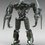# Fallacies in mathematics

Mathematicians can be wrong at times and and sometimes this errors lead to interesting results.And sometimes is some intentional effort to find foolproof solutions and really some outstanding proofs that really amaze people by unbelievable conclusions and try to show that mathematics is pointless(no,,actually not coz every fallacy is based upon some error which only a good mathematican can notice).This is what is meant by fallacies

So under this discussion u can share some of fallacies u know or mayb u hav derived urself........ Some of the fallacies i know of till date are:-

1. The fallacy of the isosceles triangle.

2. The fallacy of the right angle.

3. The fallacy that 4 = o.

4. The fallacy that +1 = -1.

5. The fallacy that all lengths are equal.

6. The fallacy that the sum of the Squares on two sides of a triangle is never less than the square on the third.

7.The fallacy that every 2 consecutive numbers are equal

8.The fallacy of the radius of a circle is indeterminate

1. The fallacy that the four points of intersection of two conics are collinear.

and many more....list is endless...in every branch of mathematics really learning some mathematical fallacies is fun....u can share someNote by Somesh Rout
7 years, 2 months ago

This discussion board is a place to discuss our Daily Challenges and the math and science related to those challenges. Explanations are more than just a solution — they should explain the steps and thinking strategies that you used to obtain the solution. Comments should further the discussion of math and science.

When posting on Brilliant:

• Use the emojis to react to an explanation, whether you're congratulating a job well done , or just really confused .
• Ask specific questions about the challenge or the steps in somebody's explanation. Well-posed questions can add a lot to the discussion, but posting "I don't understand!" doesn't help anyone.
• Try to contribute something new to the discussion, whether it is an extension, generalization or other idea related to the challenge.

MarkdownAppears as
*italics* or _italics_ italics
**bold** or __bold__ bold
- bulleted- list
• bulleted
• list
1. numbered2. list
1. numbered
2. list
Note: you must add a full line of space before and after lists for them to show up correctly
paragraph 1paragraph 2

paragraph 1

paragraph 2

[example link](https://brilliant.org)example link
> This is a quote
This is a quote
    # I indented these lines
# 4 spaces, and now they show
# up as a code block.

print "hello world"
# I indented these lines
# 4 spaces, and now they show
# up as a code block.

print "hello world"
MathAppears as
Remember to wrap math in $$ ... $$ or $ ... $ to ensure proper formatting.
2 \times 3 $2 \times 3$
2^{34} $2^{34}$
a_{i-1} $a_{i-1}$
\frac{2}{3} $\frac{2}{3}$
\sqrt{2} $\sqrt{2}$
\sum_{i=1}^3 $\sum_{i=1}^3$
\sin \theta $\sin \theta$
\boxed{123} $\boxed{123}$

Sort by:

All fallacies seem to be of immense astonishment filled with humor, but it is often shown to be violating some or the other basic mathematical rules, axioms, postulates and principles.

- 7 years, 2 months ago

true but sometimes hard to spot for amateurs

- 7 years, 2 months ago